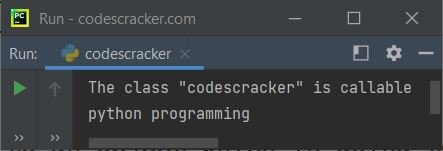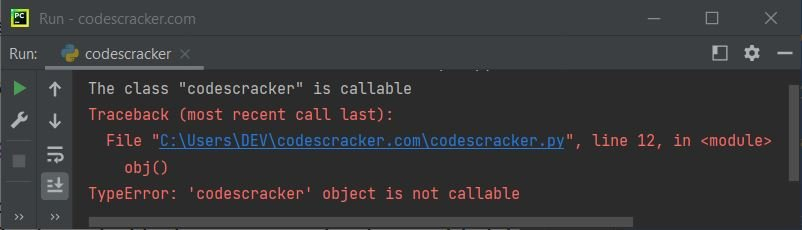# Python callable() Function

The callable() function in Python returns True if the specified object appears to be callable, otherwise returns False if the specified object is not callable. For example:

```def myf():
num = 10

print(callable(myf))

val = 20
print(callable(val))```

The output produced by this Python program, demonstrating the callable() function is:

```True
False```

Note: If callable() returns True for specified object. Then call to that object may fails. But if callable() returns False for specified object. Then call to that object will surely fail.

## Python callable() Function Syntax

The syntax of callable() function in Python is:

`callable(obj)`

where obj refers to an object that is going to check whether it is a callable object or not.

## Python callable() Function Example

Here is a simple example of callable() function in Python. In this program, I've created a class codescracker to check whether it is callable or not using of course, the callable() function:

```class CodesCracker:
def __call__(self):
print("python programming")

x = callable(codescracker)
if x:
print("The class \"codescracker\" is callable")

# following two statements proves, codescracker class is callable
obj = codescracker()
obj()
else:
print("The class \"codescracker\" is not callable")```

The snapshot given below shows the sample output produced by this Python program:Now let's create another program without __call__() to demonstrate that sometime the callable() returns True for a specified object that appears to be callable, but actually we can not call that object:

```class CodesCracker:
def myf(self):
print("python programming")

x = callable(codescracker)
if x:
print("The class \"codescracker\" is callable")

# Following two statements raises error
# As this time the class's instance is actually not callable
obj = codescracker()
obj()
else:
print("The class \"codescracker\" is not callable")```

If you execute this program, then the output would be:Because using the statement:

`x = callable(codescracker)`

The method callable() here returns True. Therefore True gets initialized to x. And because x is equal to True, therefore the condition if x or if True evaluates to be True. And the program flow goes inside the if block. And the statement:

`print("The class \"codescracker\" is callable")`

gets executed. So that we're seeing:

`The class "codescracker" is callable`

on the output before the error message. But while using the following two statements:

```obj = codescracker()
obj()```

actually using the last statement, an exception named TypeError is raised. Therefore don't believe on the callable() function only. That is, if it returns True, then do not assume that the object is actually callable. But still, you can catch the exception using the except block by putting the above two statements (or the last statement, the statement used to call) inside the try block as shown in the program given below:

```class CodesCracker:
def myf(self):
print("python programming")

x = callable(codescracker)
if x:
print("The class \"codescracker\" appears to be callable")
obj = codescracker()
try:
obj()
except TypeError:
print("But actually is not callable")
else:
print("The class \"codescracker\" is not callable")```

Now the output produced by this program is shown in the snapshot given below:That is, if the program does not raises an exception after executing the statement inside the try block, means that the object is surely callable. Therefore you can put all the things when the object is callable inside the try block.

Python Online Test

« Previous Function Next Function »